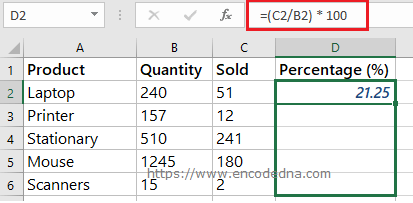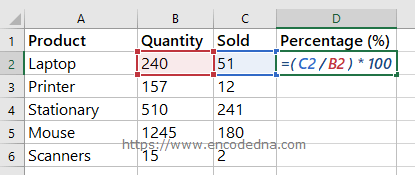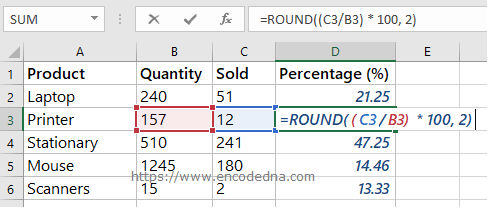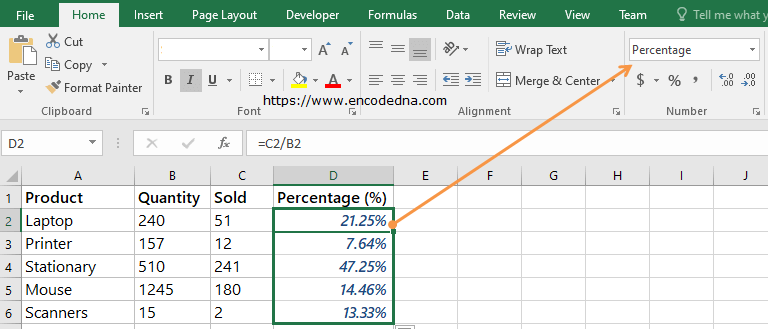﻿ Formula to Calculate Sales Percentage in Excel

# Formula to Calculate Sales Percentage in Excel

There's a simple division formula that you can use to calculate the sales percentage in Excel. Let us assume you have a list of items and number of items sold in a given period. Now, you want to calculate the sales percentage of the items.Syntax

Items_sold / total_items

See the image above. I have a list of items (or products), the total quantity and items sold within a given period. The last column shows sales percentage, which tells me the percentage of sales done for each item.

## The formulaSee the percentage column in the image. The formula to calculate the sales percentage is (sold / quantity) * 100. That is, it will first divide the value and later multiply by 100.

To apply the formula,

• select the cell D2
• insert the formula by typing =(c1 / b2) * 100
• press the enter key

Don’t have to write the formula again for other products. Simply, select cell D2, hover the mouse over the cell, you will see a little + sign, hold it and drag it down. See the image.To round of the percentage result, you can use the ROUND() function.You can further simplify the above formula. Don’t multiply the result with 100. Simply, format the cell(s) as Percentage. See the image.No need to round of the result and in-addition, it automatically adds the % symbol to the result.